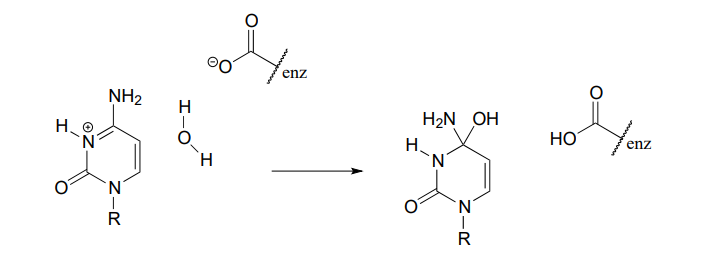# 5.6: Overview of Organic Reactivity (Problems)

$$\newcommand{\vecs}{\overset { \rightharpoonup} {\mathbf{#1}} }$$ $$\newcommand{\vecd}{\overset{-\!-\!\rightharpoonup}{\vphantom{a}\smash {#1}}}$$$$\newcommand{\id}{\mathrm{id}}$$ $$\newcommand{\Span}{\mathrm{span}}$$ $$\newcommand{\kernel}{\mathrm{null}\,}$$ $$\newcommand{\range}{\mathrm{range}\,}$$ $$\newcommand{\RealPart}{\mathrm{Re}}$$ $$\newcommand{\ImaginaryPart}{\mathrm{Im}}$$ $$\newcommand{\Argument}{\mathrm{Arg}}$$ $$\newcommand{\norm}{\| #1 \|}$$ $$\newcommand{\inner}{\langle #1, #2 \rangle}$$ $$\newcommand{\Span}{\mathrm{span}}$$ $$\newcommand{\id}{\mathrm{id}}$$ $$\newcommand{\Span}{\mathrm{span}}$$ $$\newcommand{\kernel}{\mathrm{null}\,}$$ $$\newcommand{\range}{\mathrm{range}\,}$$ $$\newcommand{\RealPart}{\mathrm{Re}}$$ $$\newcommand{\ImaginaryPart}{\mathrm{Im}}$$ $$\newcommand{\Argument}{\mathrm{Arg}}$$ $$\newcommand{\norm}{\| #1 \|}$$ $$\newcommand{\inner}{\langle #1, #2 \rangle}$$ $$\newcommand{\Span}{\mathrm{span}}$$

P5.1: For each of the nucleophilic substitution reactions below, identify the atoms which are acting as nucleophile, electrophile, and leaving group, and draw a curved-arrow diagram showing a one-step mechanism.

1.2.3.4.P5.2: Below is a reaction coordinate diagram for a hypothetical reaction.

1. What can you say about the value of $$\ce{K_{eq}}$$ for the overall A to D transformation?
2. What is the rate determining step for the overall A to D transformation?
3. Which step is faster, A to B or B to C?
4. Which transformation is more thermodynamically favorable, A to B or C to D?1. e) Below is a diagram of a hypothetical reaction. Step 2 is the rate-determining step, C is the least stable species, B is higher energy than D, and the overall reaction has an equilibrium constant $$\ce{K_{eq} = 0.33}$$. Draw a diagram that corresponds to all of this information.P5.3: Illustrated below are individual steps in some biochemical reaction mechanisms that we will be studying later. For each step, draw the products or intermediate species that would form according to the electron-movement arrows given. Be sure to include all formal charges. You do not need to show stereochemistry.

1.2.3.4.5.P5.4: Shown below are individual steps in some biochemical reaction mechanisms that we will be studying later. For each, draw curved arrows showing the electron movement taking place.

1.2.3.P5.5:

1. In the biochemical nucleophilic substitution reactions illustrated below, identify the atoms which are acting as nucleophile, electrophile, and leaving group.

Reaction 1:Reaction 2:1. Using appropriate 'R' abbreviations for regions of the molecules that are not directly involved in bond-breaking or bond-forming events above, draw curved arrows showing the electron movement that takes place in each step. Assume that both are one-step mechanisms.

## Contributors

5.6: Overview of Organic Reactivity (Problems) is shared under a CC BY-NC-SA license and was authored, remixed, and/or curated by Tim Soderberg.